# Vector equations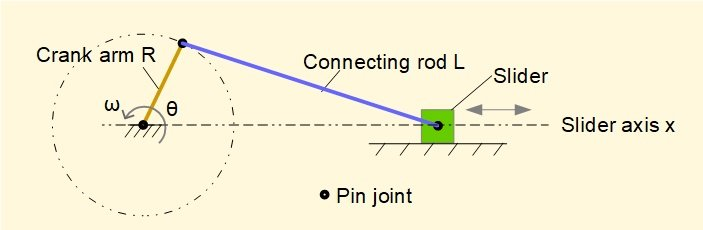In two previous tutorials in this series we obtained values for velocities and accelerations in the slider and crank mechanism by the following methods:

• from general expressions obtained by differentiating linear displacement of slider point B with respect to time (differentiating once for velocity and twice for acceleration).
• from velocity diagrams, velocity pole diagrams and acceleration diagrams, by scaling directly or by calculation from the geometry.

A disadvantage of velocity and acceleration diagrams is that each "snapshot" of the mechanism  at a specific crank angle requires a unique diagram.  By using vectors we can develop general equations to overcome this limitation.

To recap from a previous tutorial we show below how relative velocities (and accelerations) are expressed as vectors.  This relationship is the basis of the vector equations that follow.

Note that in this tutorial we change the convention previously used to designate quantities.  Quantities denoted by a single subscript are assumed to be relative to "ground" frame of reference point O.   Thus in this example va/o is designated va. Relative quantities are denoted as before with a dual subscript, for example vb/a is the velocity of point B relative to point A.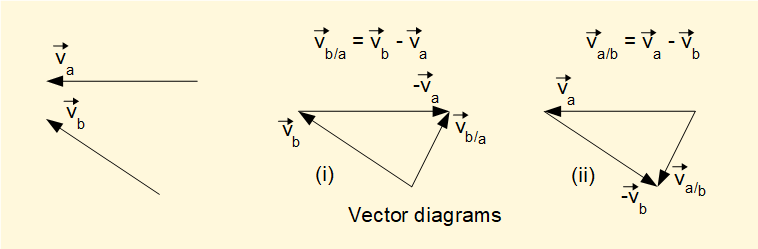We now develop vector equations for velocity and acceleration for a generic crank mechanism shown below.  In both cases the angular velocity ω of the crank arm is constant.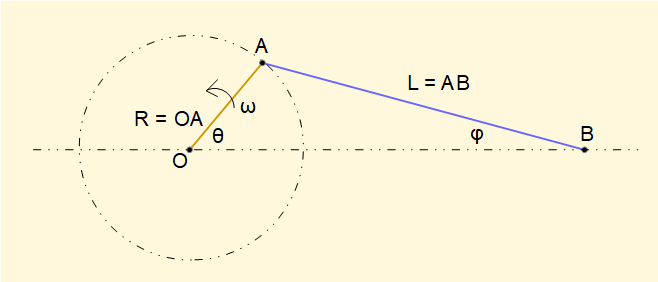#### Velocities

The diagram below shows the velocity vectors with known directions.  If derivation of these vectors is unclear refer to the previous tutorial.  Note the directions chosen for unit vectors i and j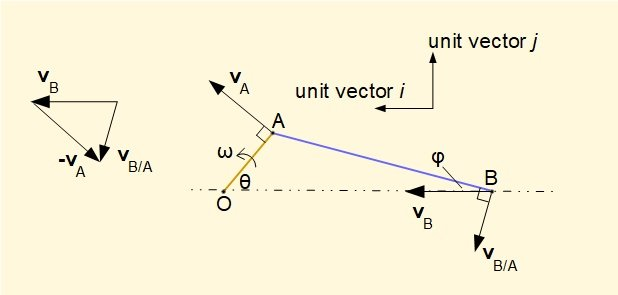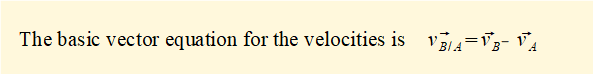We can express vA, vB and vB/A  in terms of unit vectors i and j as follows (taking particular note of signs according to the defined directions of unit vectors).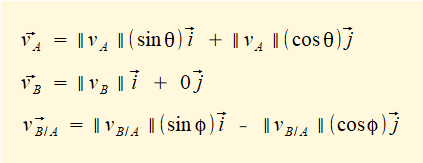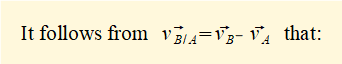From the above we now develop equations for scalar values of velocities vB/A and vB for all crank angles θ using expressions for sin φ  and cos φ in terms of sin θ and cos θ derived in a previous tutorial, viz.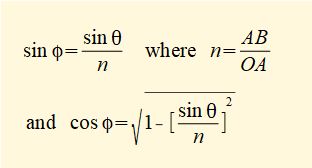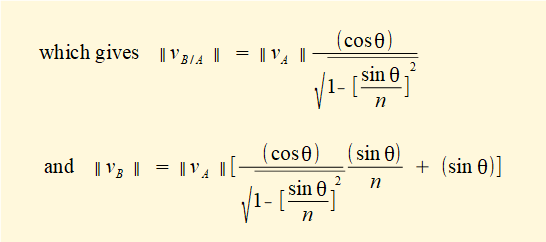We now apply these equations to the crank mechanism with parameters stated below (we used this example in previous tutorials).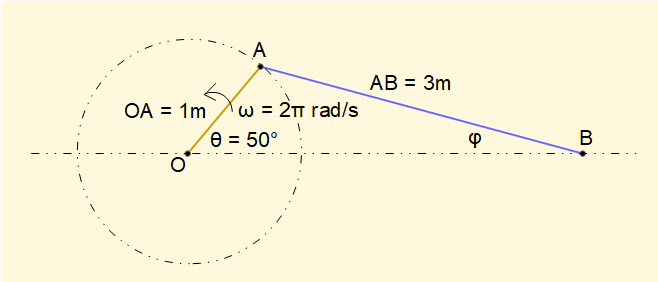||vA|| =  ω (OA) = (2π)(1) = 2π m/s

n = AB/OA = 3

The plot below shows ||vB|| against crank angle θ.It can be shown that the expression for vB obtained above is equivalent to the one obtained in a previous tutorial by differentiation of displacement of point B with respect to time, viz.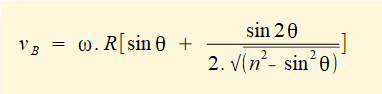The plot below shows the angular velocity ωAB  of the connecting rod AB against crank angle θ from the relation ωAB  = ||vB/A||/(AB) with ||vB/A|| computed from the above expression.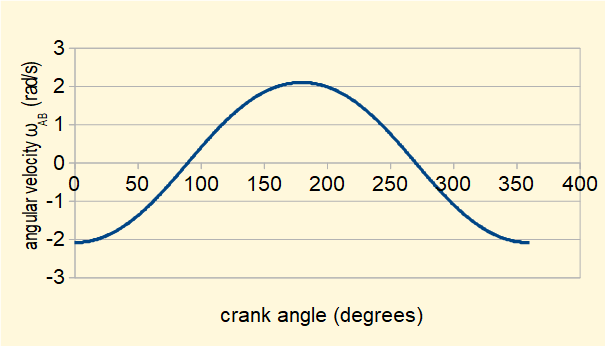This plot shows the sign of ωAB changes at θ = 90° and θ = 270° during one revolution of the crank arm.  The sign convention follows the right-hand rule for vector cross-product vt ω x r (where vt is the tangential velocity).  In this convention anti-clockwise rotations are positive and clockwise rotations are negative.  r is the position vector of the rotating point relative to its centre of rotation, in this case point B rotating about point A.  The diagrams below illustrate the directions of ωAB  and vB/A in each quadrant of rotation of the crank arm.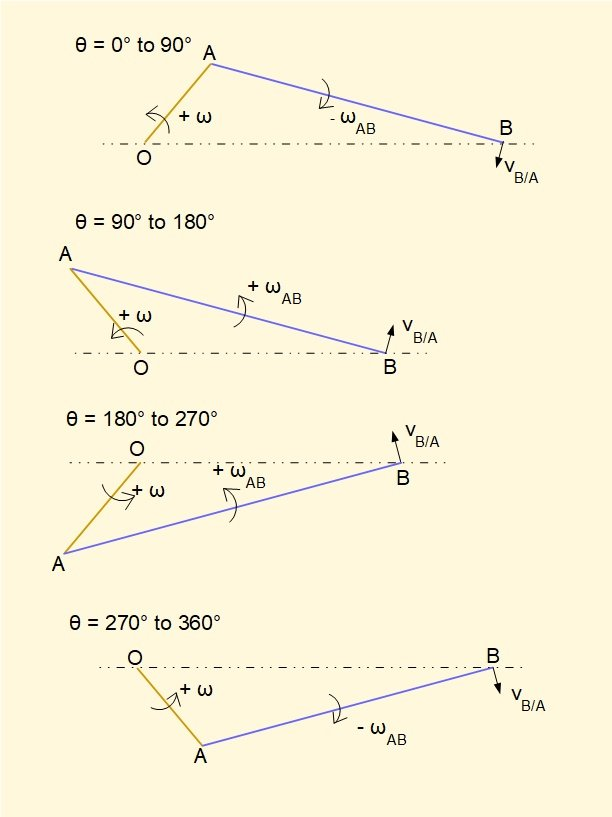#### Accelerations

The diagram below shows the acceleration vectors with known directions. Note that aB/A has two components, radial component arB/A directed towards centre of rotation A and tangential component atB/A.  If derivation of these vectors is unclear refer to the previous tutorial.  Note the directions chosen for unit vectors i and j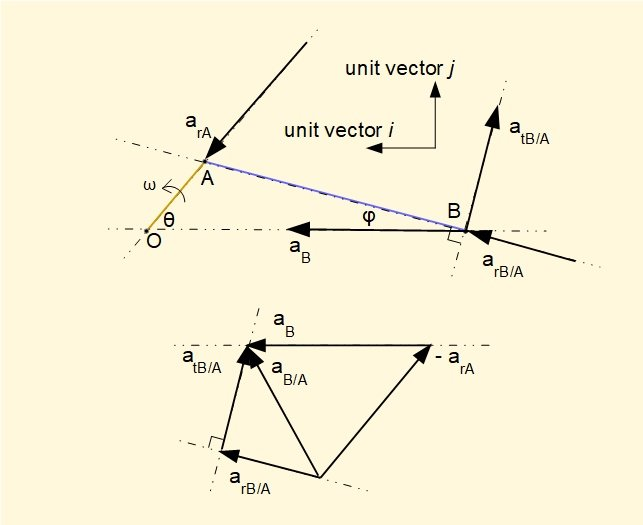To determine direction of vector atB/A  we examine the plot for ωAB  (see above).  We have drawn the vector diagram with crank angle θ in the first quadrant where the plot shows ωAB (and hence vtB/A) decreasing in magnitude. Thus atB/A must oppose the direction of vtB/A

However, we cannot assign the direction of aB with certainty.  As this is the only acceleration vector in the diagram with its direction undetermined we assign an arbitrary direction of positive unit vector i and allow the equations to determine direction as seen below.

It should be noted that the vector diagram above can be constructed for any crank angle θ crank cycle with corresponding lines of action and directions of vectors.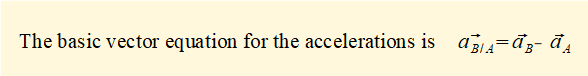We can express aA, aB and aB/A  in terms of unit vectors i and j as follows (taking particular note of signs according to the defined direction of unit vectors).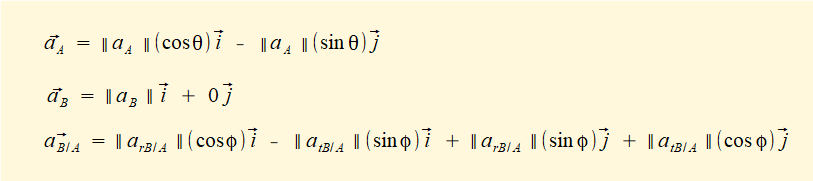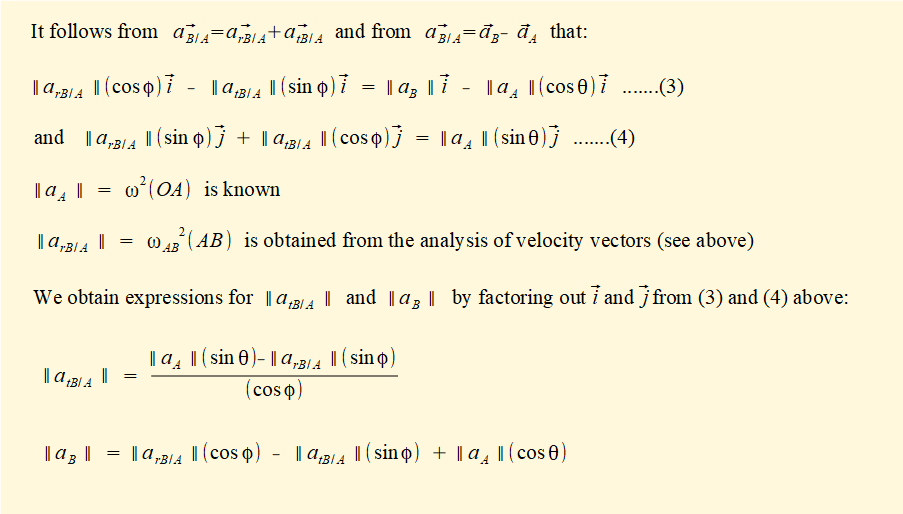It can be shown that the expression for ||aB || above is equivalent to one obtained in a previous tutorial by differentiation of velocity vB, viz.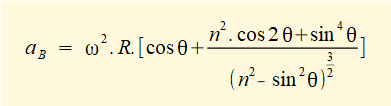We compute ||aB || and ||atB/A ||  for the example mechanism below expressing sin φ  and cos φ in terms of sin θ and cos θ as before, viz.The plot below shows ||aB||. It is identical to the plot in a previous tutorial obtained by sequential differentiation of displacement of point B with respect to time.The two plots below show respectively ||atB/A||  the tangential acceleration of point B relative to point A and angular acceleration  αAB  the relationship between the two quantities being  ||atB/A|| = αAB  |AB|  This relationship in general terms is the vector cross-product at = α x r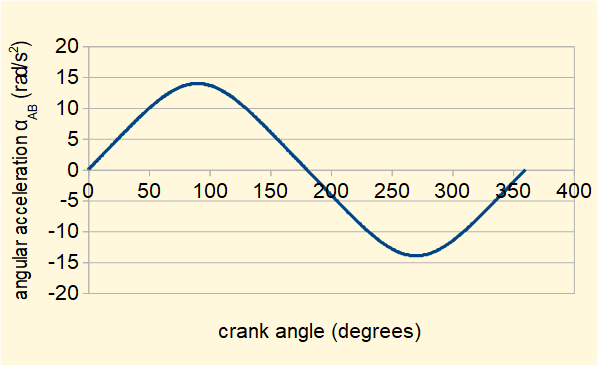It is instructive to compare the plots of αAB  above and ωAB  below. The relationships are best illustrated in the second group of diagrams below where validity of vector cross-products vt ω x r   and at = α x r  is confirmed remembering that vector r is the position vector of point B relative to point A.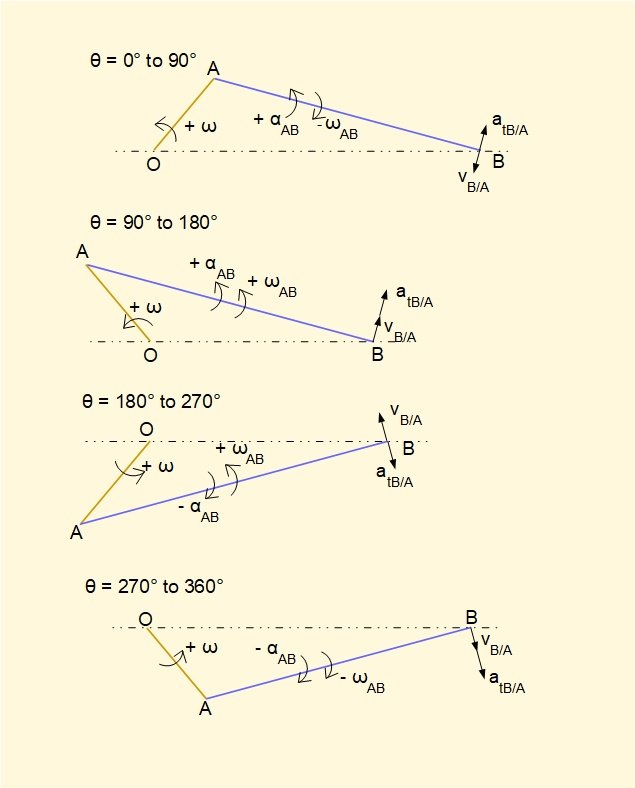The table below provides an overview of crank angles θ where values of kinematic parameters are zero, positive maximum and negative maximum.

 Value of parameter vB aB ωAB αAB zero θ = 0 ° and 180° θ identical to maximum values of vB θ = 90° and 270° θ = 0° and 180° maximum (positive) θ <  90° dependent on ratio n θ = 0 ° θ = 180° θ = 90° maximum (negative) θ > 270° dependent on ratio n two symmetrical points θ <  180° and θ  >  180° dependent on ratio n θ = 0° θ = 270°

I welcome feedback at:

### Tutorials - mechanical vibrations

###### Forced vibrations with damping

Alistair's tutorials 2020